Home | | Maths 8th Std | Try these, Recap Exercise, Student Activities, Think and answer

# Try these, Recap Exercise, Student Activities, Think and answer

8th Maths : Chapter 5 : Geometry : Try these, Recap Exercise, Student Activities, Think and answer - Numerical Example solved problems and Numerical problems Questions with Solution

Answer the following questions by recalling the properties of triangles:

1. The sum of the three angles of a triangle is _____________ . [Answer: 180°]

2. The exterior angle of a triangle is equal to the sum of the ____________ angles to it. [Answer: interior]

3. In a triangle, the sum of any two sides is ____________ than the third side. [Answer: greater]

4. Angles opposite to equal sides are _______ and vice – versa. [Answer: Equal]

5. What is A in the triangle ABC?Solution:

The exterior angle = sum of interior opposite angles.

∴ ∠A +  C = 150° in ΔABC

But  C = 40° [∵ Vertically opposite angler are equal]

∴ ∠A +  C = 150°

=>  A +  40° = 150°

A = 150° − 40°

A = 110°

Try these

Identify the pairs of figures which are similar and congruent and write the letter pairs.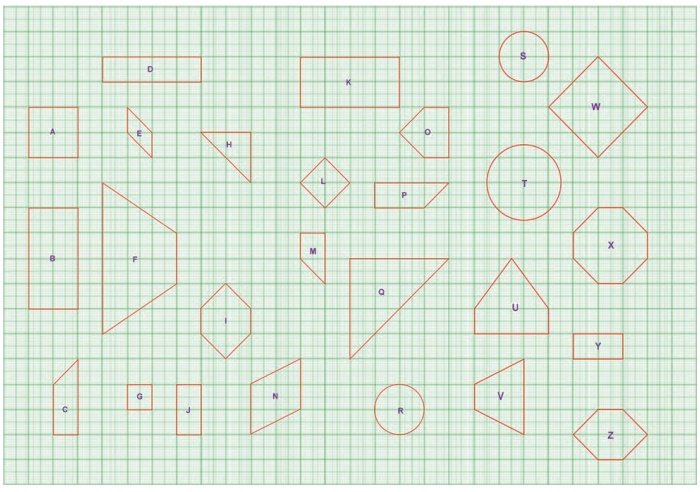Solution:

Similar shapes:

(i) W and L

(ii) B and J

(iii) A and G

(iv) B and J

(v) B and Y

(vi) E and N

(vii) H and Q

(viii) R and T

(ix) S and T

Congruent shapes:

(i) Z and I

(ii) J and Y

(iii) C and P

(iv) B and K

(v) R and S

(vi) I and Z

You can find more.

Try these

Match the following by their congruence propertyAnswer: 1. (iv), 2. (iii), 3. (i), 4. (ii)

Think

In the figure, DA = DC and BA = BC.

Are the triangles DBA and DBC congruent? Why?Solution:

AB = CB

DB = DB (common)

ΔDBA = ΔDBC        [ By SSS congruency]

Also RHS rule also bind here to say their congruency.

Activity

The teacher cuts many triangles that are similar or congruent from a card board (or) chart sheet. The students are asked to find which pair of triangles are similar or congruent based on the measures indicated in the triangles.

Activity

1. We can construct sets of Pythagorean triplets as follows.

Let m and n be any two positive integers (m > n):

(a, b, c) is a Pythagorean triplet if a = m2  n2 , b = 2mn and c = m2 + n2 (Think, why?)

Complete the table.2. Find all integer-sided right angled triangles with hypotenuse 85.

Solution:

(x + y)2 − 2xy = 852Pythagorean triplets with hypotenuse 85.

Activity

1. Consider a paper cut-out of a triangle. (Let us have an acute-angled triangle, to start with). Name it, say ABC.2. Fold the paper along the line that passes through the point A and meets the line BC such that point B falls on C. Make a crease and unfold the sheet.3. Mark the mid point M of BC.4. You can now draw the median AM, if  you want to see it clearly. (Or you can  leave it as a fold).5. In the same way, fold and draw the other two medians.6. Do the medians pass through the same point?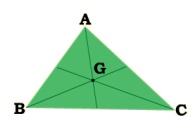Now you can repeat this activity for an obtuse - angled triangle and a right triangle.

What is the conclusion? We see that,

The three medians of any triangle are concurrent.

Activity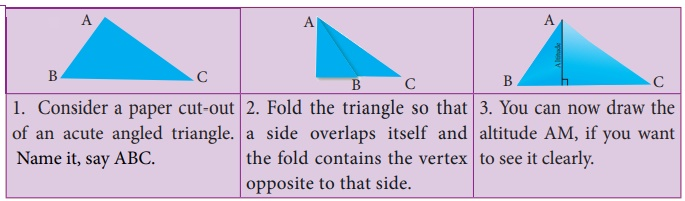In the same way, you find altitudes of other two sides. Also, with the help of your teacher, you find altitudes of right angled triangle and obtuse angled triangle. Do the altitudes of triangle pass through the same point? What is your conclusion? We see that,

The three altitudes of any triangle are concurrent.

The point of concurrence is known as its Orthocentre, denoted by the letter H.

Think

1. In any acute angled triangle, all three altitudes are inside the triangle. Where will be the orthocentre?

In the interior of the triangle or in its exterior?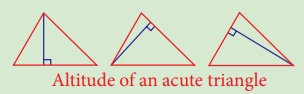Solution: Interior of the triangle.

Altitude of an acute triangle

2. In any right angled triangle, the altitude perpendicular to the hypotenuse is inside the triangle; the other two altitudes are the legs of the triangle. Can you identify the orthocentre in this case?Solution: Vertex containing 90°

Altitude of a right triangle

3. In any obtuse angled triangle, the altitude connected to the obtuse vertex is inside the triangle, and the two altitudes connected to the acute vertices are outside the triangle. Can you identify the orthocentre in this case?Solution: Exterior of the triangle.

Altitude of an obtuse triangle

Activity

One can visualize the point of concurrence of the perpendicular bisectors, through simple paper folding. Try and see that,

The perpendicular bisectors of the sides of any triangle are concurrent.As done in the earlier activity on Centroid, you can repeat the experiment for various types of triangle, acute, obtuse, right, isosceles and equilateral. Do you find anything special with the equilateral triangle in this case?

Activity

Check if the following are true by paper-folding:

• The circumcentre of an acute angled triangle lies in the interior of the triangle.

• The circumcentre of an obtuse angled triangle lies in the exterior of the triangle.

• The circumcentre of a right triangle lies at the midpoint of its hypotenuse.

Activity

1. Consider a paper cut-out of a triangle. Name it, say ABC.2. Fold the triangle so that the opposite sides meet and contain the vertex. Repeat the same to find angle bisectors of other two angles also.3. Trace all of the folds.

Do the angle bisectors pass through the same point?Now you can repeat this activity for an obtuse-angled triangle and a right angled triangle. What is the conclusion? Do the angle bisectors pass through the same point in all the cases? Yes, we see that,

The three angle bisectors of any triangle are concurrent.

Try these

Identify the type of segment required in each  triangle:

(median, altitude, perpendicular bisector, angle bisector)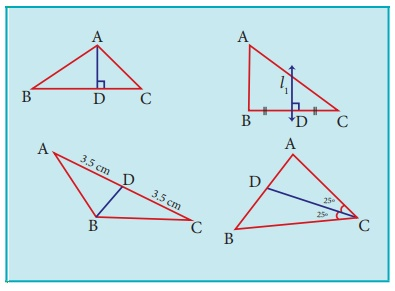(i) AD = Altitude (ii) l1 = Perpendicular bisector

(iii) BD = Median (iv) CD = Angular bisector

Activity

1. By paper folding, find the centroid, orthocentre, circumcentre and incentre of an equilateral triangle. Do they coinside?

2. By paper folding, find the centroid (G), orthocentre(H), circumcentre (S) and incentre (I) of a triangle. Join G,H,S and I. Are they collinear?

Think

Is it possible to construct a quadrilateral PQRS with PQ = 5 cmQR = 3 cmRS = 6 cmPS = 7 cm and PR = 10 cm. If not, why?

Solution:The lower triangle cannot be constructed as the sum of two sides

5 + 3 = 8 < 10 cm. So this quadrilateral cannot be constructed.

Try these

1. The area of the trapezium is _________.  [Answer: 1/2 × h (a + b) sq. units]

2. The distance between the parallel sides of a trapezium is called as _________. [Answer: its height]

3. If the height and parallel sides of a trapezium are 5cm, 7cm and 5cm respectively, then its area is _________. [Answer: 30 sq cm ]

Solution:

= 1/2 × h × (a + b) sq. units

= 1/2 × 5 × (7 + 5) = 1/2 × 5 × 12 = 30 sq.cm

4. In an isosceles trapezium, the non-parallel sides are _________ in length. [Answer: equal]

5. To construct a trapezium, _________ measurements are enough. [Answer: four]

6. If the area and sum of the parallel sides are 60 cm2 and 12 cm , its height is _________. [Answer: 10 cm]

Solution:

Area of the trapezium = 1/2 × h (a + b)

60 = 1/2 × h (12)

h = [60 × 2] / 12 = 10 cm

Activity

1. Place a pair of unequal sticks (say pieces of broomstick) such that they have their end points joined at one end.2. Now place another such pair meeting the free ends of the first pair.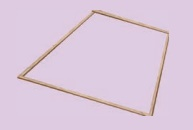What is the figure enclosed? It is a quadrilateral. Name it as ABCD. How many sides are there? What are its diagonals? Are the diagonals equal? Are the angles equal?

In the above activity can you get a quadrilateral in which

(i) All the four angles are acute.

(ii) One of the angles is obtuse.

(iii) Two of the angles are obtuse.

(iv) One of the angles is a right angle.

(v) Two of the angles are right angles.

(vi) The diagonals are mutually r.

Activity

1. A pair of identical 30º–60º –90º set-squares are needed for this activity. Place them as shown in the figure.(i) What is the shape we get? It is a parallelogram.

(ii) Are the opposite sides parallel? − Answer: Yes

(iii) Are the opposite sides equal? − Answer: Yes

(iv) Are the diagonals equal? − Answer: No

(v) Can you get this shape by using any other pair of identical set-squares? − Answer: Yes

2. We need a pair of 30º–60º –90º  set- squares for this activity. Place them as shown in the figure.(i) What is the shape we get? − Answer: Rectangle

(ii) Is it a parallelogram? Answer: Yes

It is a quadrilateral; infact it is a rectangle. (How?) Answer: Opposits sides are equal.  All angles = 90°

(iii) What can we say about its lengths of sides, angles and diagonals?

Discuss and list them out.

Opposite sides are equal

All angles are equat and are = 90°

Diagonals are equal

3. Repeat the above activity, this time with a pair of 45º–45º–90º set-squares.(i) How does the figure change now? Is it a parallelogram? It becomes a square! (How did it happen?)

Answer: All sides are equal. So it is square.

(ii) What can we say about its lengths of sides, angles and diagonals?

Discuss and list them out.

All sides are equal

All angles = 90°

Diagonals equal

(iii) How does it dier from the list we prepared for the rectangle?

All sides are equal.

Diagonals bisects each other.

4. We again use four identical 30º –60º –90º set- squares for this activity. Note carefully how they are placed touching one another.(i) Do we get a parallelogram now? Answer: Yes

(ii) What can we say about its lengths of sides, angles and diagonals?

All sides equal.

opposite sides are equal and parallel.

(iii) What is special about their diagonals?

Try these

1. Say True or False:

(a) A square is a special rectangle. [Answer: True]

(b) A square is a parallelogram. [Answer: True]

(c) A square is a special rhombus. [Answer: True]

(d) A rectangle is a parallelogram [Answer: False]

(a) which have diagonals bisecting each other.

(b) In which the diagonals are perpendicular bisectors of each other.

(c) Which have diagonals of dierent lengths.

(d) Which have equal diagonals.

(e) Which have parallel opposite sides.

(f) In which opposite angles are equal.

3. Two sticks are placed on a ruled sheet as shown. What figure is formed if the four corners of the sticks are joined?

(a) Two unequal sticks. Placed such that their midpoints coincide.(b) Two equal sticks. Placed such that their midpoints coincide.(c) Two unequal sticks. Placed intersecting at mid points perpendicularly.(d) Two equal sticks. Placed intersecting at mid points perpendicularly.(e) Two unequal sticks. Tops are not on the same ruling. Bottoms on the same ruling. Not cutting at the mid point of either.(f) Two unequal sticks. Tops on the same ruling. Bottoms on the same ruling. Not necessarily cutting at the mid point of either.(a) parallelogram

(b) Rectangle

(c) Rhombus

(d) Square

(f) Trapezium

Note

• Any segment or angle is congruent to itself! This is called Reflexive property

• If two triangles are congruent, then their corresponding parts are congruent. This is called CPCTC (Corresponding parts of Congruent Triangles are Congruent).

• If angles then sides means if two angles are equal in a triangle, then the sides opposite to them are equal.

• If sides then angles means if two sides are equal in a triangle, then the angles opposite to them are equal.

SSA and ASS properties are not sucient to prove that two triangles are congruent. This is explained in the given figure. By construction, in triangles ABD and ABC, BC = BD = a. Also, AB and BAZ are common. But AC  AD. So, ∆ABD is not congruent to ∆ABC and so SSA fails.Note

• All circles and squares are similar to each other.

• Not all rectangles need to be similar always.

• If two angles are both congruent and supplementary then, they are right angles.

• All congruent triangles are similar.

• The symbol ~ is used to denote similarity.

Note

We can split any quadrilateral into two triangles by drawing a diagonal.In the above figures, a quadrilateral is split in two ways by its diagonals. So, if a diagonal is given, first draw the lower triangle with two sides and one diagonal. Then, draw the upper triangle with other two measures.

(i) • A polygon in which atleast one interior angle is more than 180°, is called a concave polygon. In the given polygon, interior angle at C is more than 180°.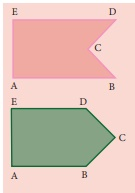• A polygon in which each interior angle is less than 180°, is called a convex polygon. In the given polygon, all interior angles are less than 180°.

(ii) Look at the following quadrilaterals.Though, we can construct a quadrilateral in two ways as shown above, we do not take into account the concave quadrilaterals in this chapter. Hence, the construction of only convex quadrilaterals are treated here.

Note

Consider the given Quadrilateral ABCD. In which AC is a diagonal (d), BE (h1) and DF (h2) are the perpendiculars drawn from the vertices B and D on diagonal AC.

Area of quadrilateral ABCD = Area of ABC + Area of ACD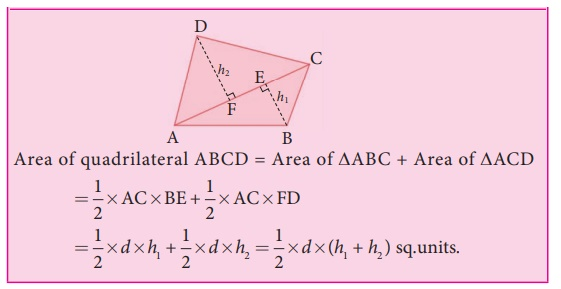Tags : Questions with Answers, Solution | Geometry | Chapter 5 | 8th Maths , 8th Maths : Chapter 5 : Geometry
Study Material, Lecturing Notes, Assignment, Reference, Wiki description explanation, brief detail
8th Maths : Chapter 5 : Geometry : Try these, Recap Exercise, Student Activities, Think and answer | Questions with Answers, Solution | Geometry | Chapter 5 | 8th Maths